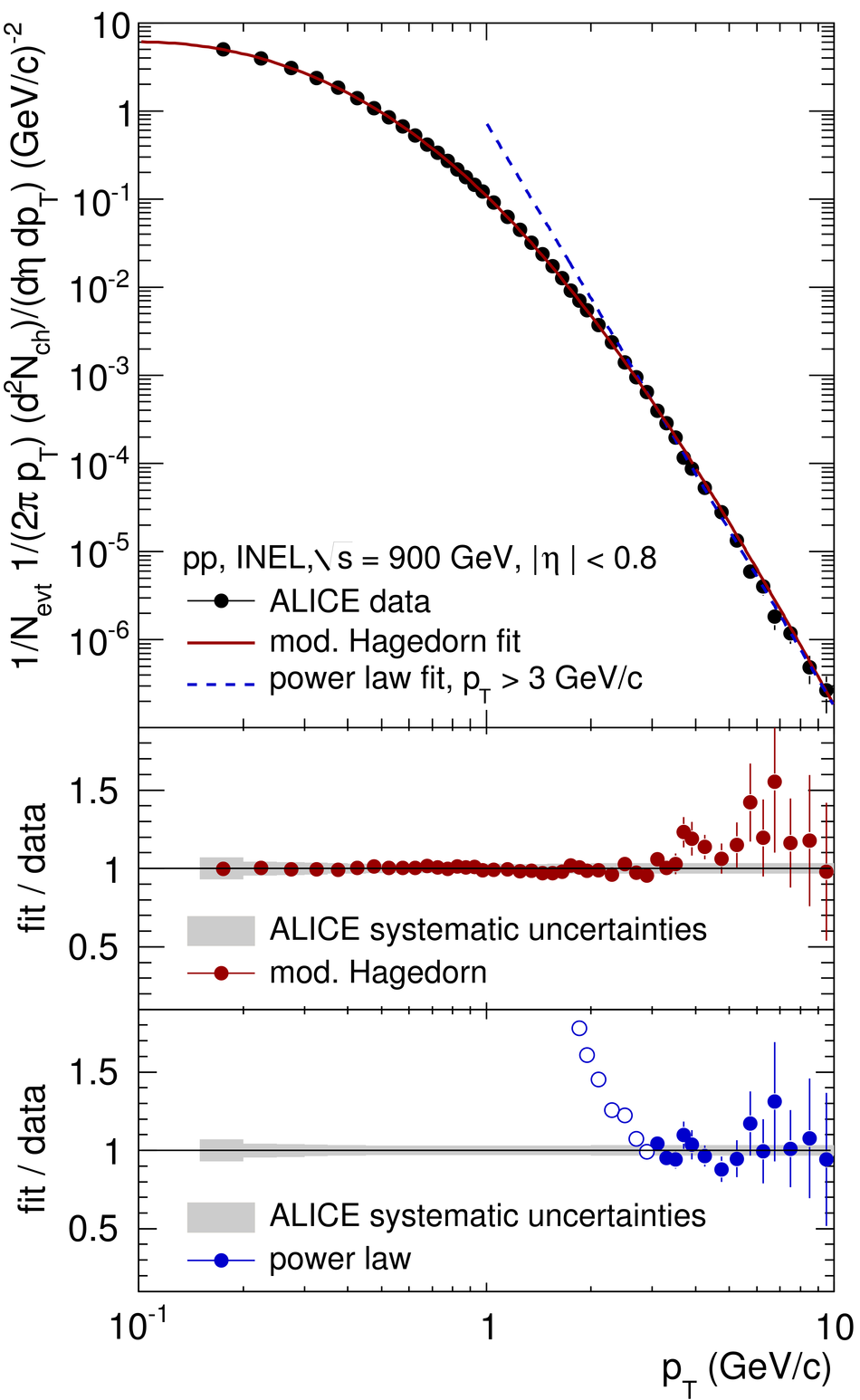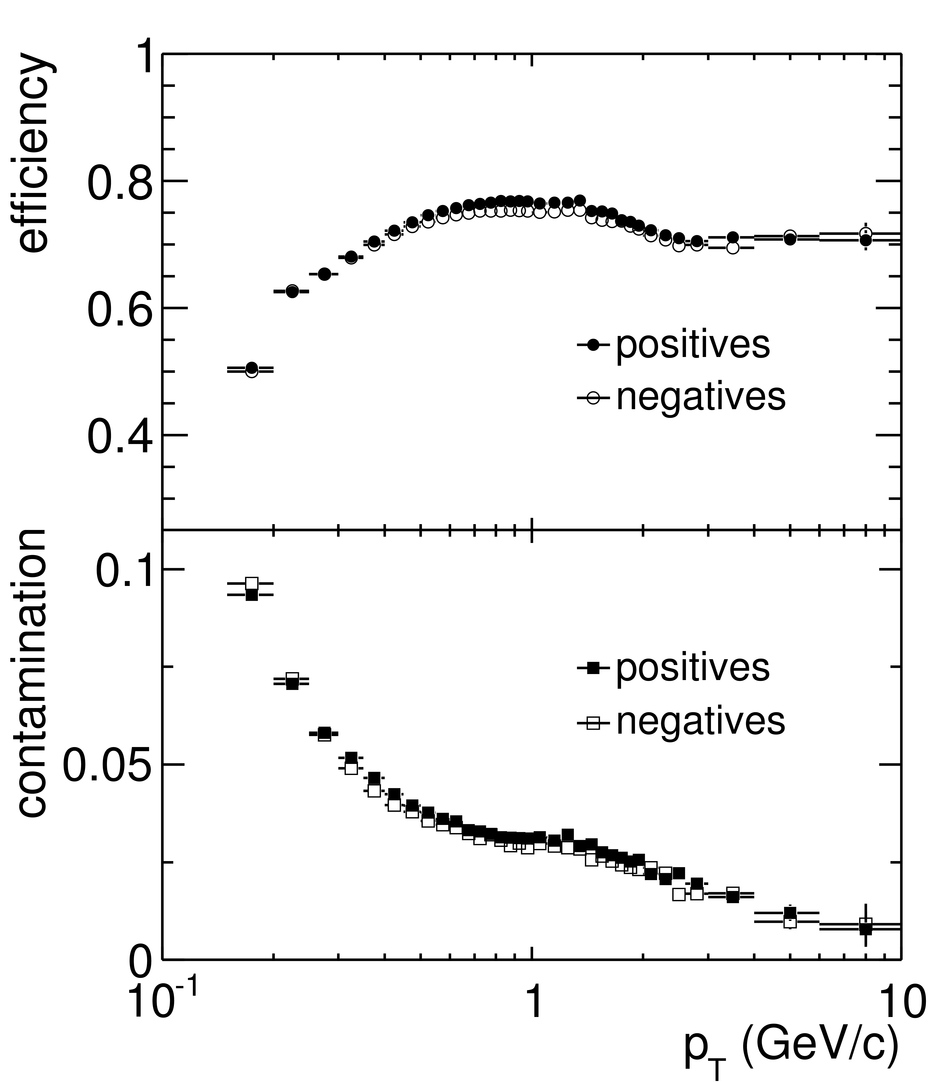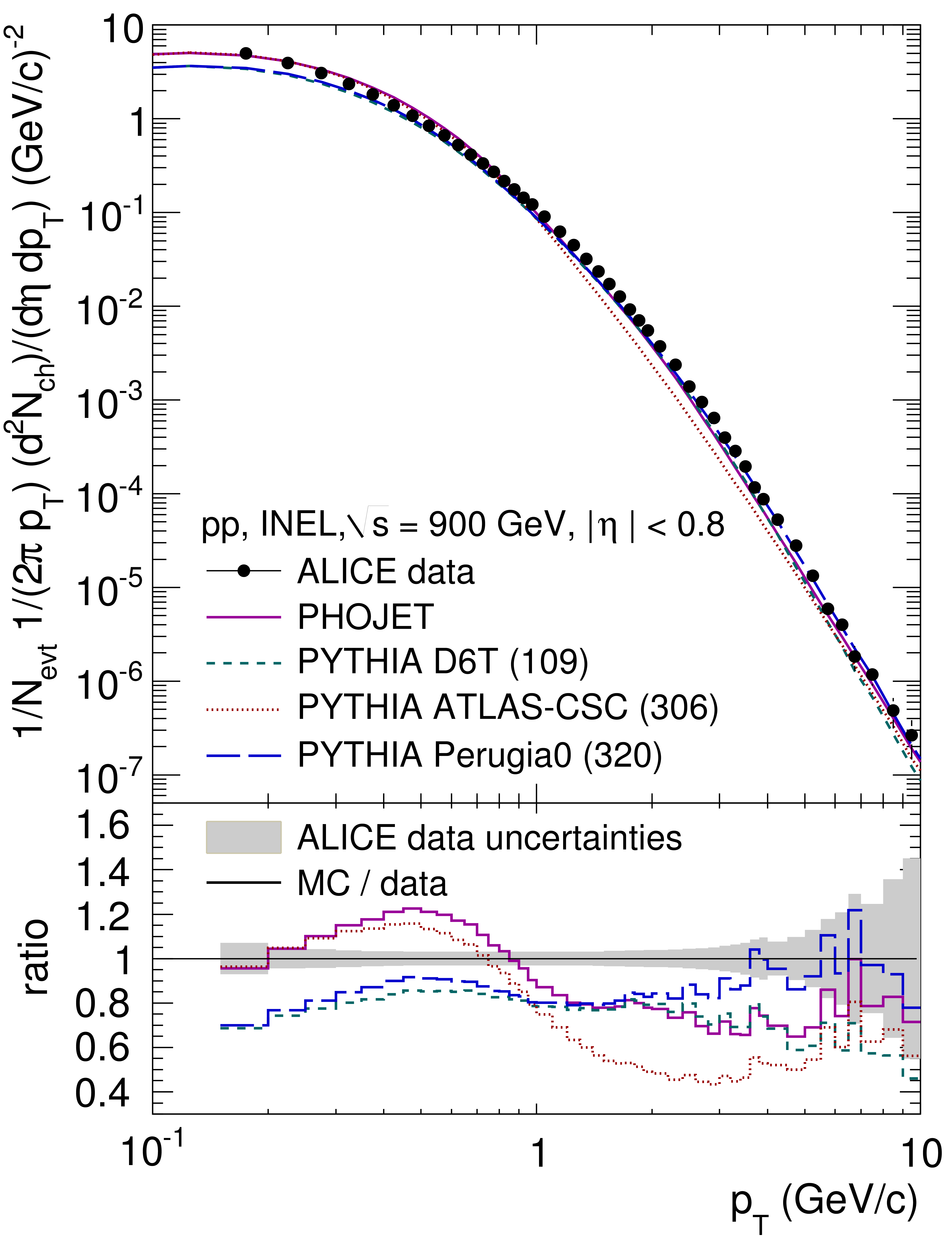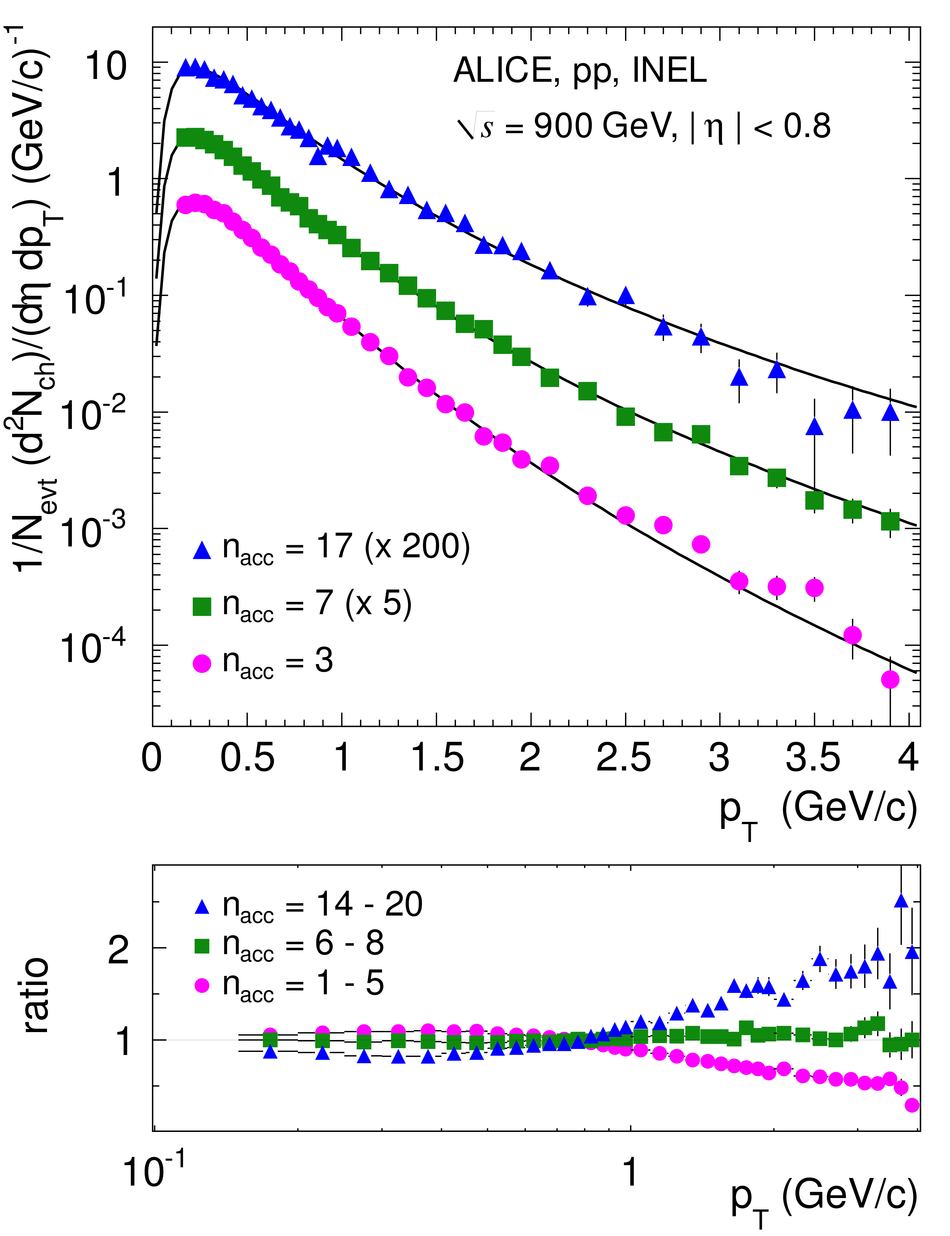# Transverse momentum spectra of charged particles in proton-proton collisions at $\sqrt{s} = 900$ GeV with ALICE at the LHC

The inclusive charged particle transverse momentum distribution is measured in proton-proton collisions at $\sqrt{s} = 900$ GeV at the LHC using the ALICE detector. The measurement is performed in the central pseudorapidity region $(|\eta|<~0.8)$ over the transverse momentum range $0.15<~p_{\rm T}<~10$ GeV/$c$. The correlation between transverse momentum and particle multiplicity is also studied. Results are presented for inelastic (INEL) and non-single-diffractive (NSD) events. The average transverse momentum for $|\eta|<~0.8$ is $\left<~p_{\rm T}\right>_{\rm INEL}=0.483\pm0.001$ (stat.) $\pm0.007$ (syst.) GeV/$c$ and $\left<~p_{\rm T}\right>_{\rm NSD}=0.489\pm0.001$ (stat.) $\pm0.007$ (syst.) GeV/$c$, respectively. The data exhibit a slightly larger $\left<~p_{\rm T}\right>$ than measurements in wider pseudorapidity intervals. The results are compared to simulations with the Monte Carlo event generators PYTHIA and PHOJET.

Figures

## Figure 2

 Normalized differential primary charged particle yield in INEL $pp$ collisions at $\sqrt{s}=900~$GeV, averaged in$|\eta|< 0.8$. The fit ranges are $0.15< p_T< 10$~GeV/$c$ for the modified Hagedorn function (Eq.1) and $3< p_T< 10~$GeV/$c$ for the power law (Eq.2) In the lower panels, the ratios fit over data are shown The open symbols indicate here data points which are not included in the fit Errors bars are statistical only. Indicated as shaded areas are the relative systematic data errors.## Figure 3

 Left panel: Normalized differential primary charged particle yield in NSD $pp$ collisions at $\sqrt{s}=900~$GeV, averaged in $|\eta|< 0.8$ The ALICE data are compared to results from ATLAS and CMS in $pp$ at the same energy [19,20] Right panel:Normalized invariant primary charged particle yield in NSD $pp$ collisions at $\sqrt{s}=900~$GeV, averaged in $|\eta|< 0.8$ The ALICE data are compared to results from UA1 in$p\bar{p}$ at the same energy . For the computation of the invariant yield, it has been assumed that all particles are pions The shaded areas indicate the statistical and systematic errors added in quadrature.## Figure 7

 The average transverse momentum of charged particles in INEL $pp$ events at $\sqrt{s}=900~$GeV for three different $p_T$ ranges as a function of $n_{\rm acc}$ (left panel) and as a function of $n_{\rm ch}$ (right panel) The error bars and shaded areas indicate the statistical and systematic errors, respectively.## Figure 8

 The average transverse momentum of charged particles for $0.5< p_T< 4~$GeV/$c$(left panel) and $0.15< p_T< 4~$GeV/$c$ (right panel) in INEL $pp$ events at$\sqrt{s}=900~$GeV as a function of $n_{\rm ch}$ in comparison to models. The error bars and the shaded area indicate the statistical and systematic errors of the data, respectively In the lower panels, the ratio Monte Carlo over data is shown The shaded areas indicate the statistical and systematic uncertainty of the data,added in quadrature.## Figure 1

 Charged-particle track reconstruction efficiency for primary particles (top) and contamination from secondary particles (bottom), for positively and negatively charged particles in $|\eta|< 0.8$ as a function of $p_T$. The tracking efficiency is normalized to the number of generated primary particles using PYTHIA. The contamination from secondary tracks was scaled in Monte Carlo to match the measured $d_0$ distributions.## Figure 4

 Energy dependence of the average transverse momentum of primary charged particles in $pp$ and $p\bar{p}$ collisions. Data from other experiments are taken [20,21,22,23,24,25].## Figure 5

 Top: Comparison of the primary charged particle differential yield in INEL $pp$ collisions at $\sqrt{s}=900~$GeV ($|\eta|< 0.8$) to results from PHOJET and PYTHIA tunes 109 , 306  and 320 . Bottom: Ratio between the Monte Carlo simulation and the data. The shaded area indicates the statistical and systematic errors of the ALICE data added in quadrature.## Figure 6

 Upper panel: Transverse momentum spectra of primary charged particles in INEL $pp$ collisions at $\sqrt{s}=900~$GeV ($|\eta|< 0.8$), normalized to the total number of INEL events $N_{\rm evt}$, for three different event multiplicities together with the modified Hagedorn fits (Eq.1) described in the text. The fits are performed in the range $0.15 < p_T < 4$~GeV/$c$ and extrapolated to $p_T=0$. The error bars indicate the statistical and systematic errors added in quadrature. Lower panel: Ratios of the $p_T$ spectra in different multiplicity ranges to the inclusive $p_T$ spectrum in INEL events.